# Pre-Algebra : Multiplicative Identity Property

## Example Questions

### Example Question #1 : Multiplicative Identity Property

What property can be applied to the following expression?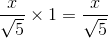Multiplicative Inverse

Multiplicative Identity

Distributive Law

Associative of Multiplication

Multiplicative Identity

Explanation:

The rule for Multiplicative Identity Property is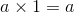Expression given in the question is:Hence the property is Multiplicative Identity.

### Example Question #2 : Multiplicative Identity Property

Which of the following demonstrates the multiplicative inverse property?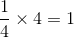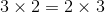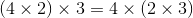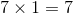Explanation:

The multiplicative inverse property states that for some value,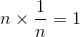.

Therefore, the statement demonstrating the multiplicative inverse property is.

### Example Question #1 : Multiplicative Identity Property

Which of the following statements demonstrates the identity property of multiplication?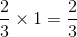None of the examples in the other responses demonstrates the identity property of multiplication.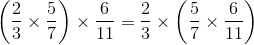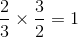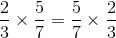Explanation:

The identity property of multiplication states that there is a number 1, called the multiplicative identity, that can be multiplied by any number to obtain that number. Of the four statements,demonstrates this property.

### Example Question #1 : Multiplicative Identity Property

Which property does the equation below represent?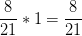Distributive Property

Zero Property of Multiplication

Associative Property of Multiplication

Communitive Property of Multiplication

Multiplicative Identity Property

Multiplicative Identity Property

Explanation:

When something is multiplied by the number one it remains the same number. Therefore when multiplying by one it creates its own identity.

Multiplicative Identity Property

### Example Question #1 : Multiplicative Identity Property

Which equation shows an example of the multiplicative identity property?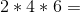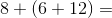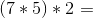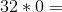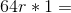Explanation:

The multiplicative identity property means that when something is multiplied by 1 then it will remain the same as the other number.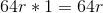### Example Question #1 : Multiplicative Identity Property

Which of the following displays the multiplicative identity property?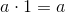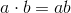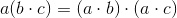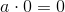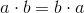Explanation:

The multiplicative identity property states that when you multiply a number by 1, the answer is the original number.

Therefore,displays the multiplicative identity property.

### All Pre-Algebra Resources